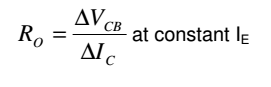## Ac output resistance in common base configuration, Physics

Assignment Help:

AC  Output  Resistance  in  Common  Base  Configuration

Ratio  of  change  in collector-base  voltage  ( ? VCB)  to  resulting  change  in  collector  current  ( ? IC)  at constant emitter current (IE). i.e.Output Resistance of CB configuration is very high, of the order of several tens of kilo-ohms (≅1 M?).

#### Expression for the intensity distribution , Q.   Derive an expression for t...

Q.   Derive an expression for the intensity distribution due to Fraunhofer's diffraction at a single slit and shows that the intensity of the first subsidiary maxima is about 4.5 p

#### Force, Define one newton

Define one newton

Hello

#### What is shiro mass, Logan, whose mass is 18 kg, sits 1.20 m from the center...

Logan, whose mass is 18 kg, sits 1.20 m from the center of a seesaw. If Shiro must sit 0.80 m from the center to balance Logan, what is Shiro's mass?

#### State boyle''s law , "At base temperature the volume of a gas differs inver...

"At base temperature the volume of a gas differs inversely with pressure". If P and V are volume and pressure of a gas at a given temperature then

#### Do glass or polymer transmits the radiation, Do glass or polymer transmits ...

Do glass or polymer transmits the radiation? There is not a simple answer since it will always depend on the last use and working conditions of the system. Usually speaking gla

#### Cube of copper , Calculate how much heat energy is required to raise the te...

Calculate how much heat energy is required to raise the temperature of a cube of copper of side 2cm. from 300 to 320k. given: density=8933kg/m cube , atomic weight=63.55, cp=24j/k/

#### Mechanics, A gas is compressed from 400 cm3 to 200 cm3 at a constant pressu...

A gas is compressed from 400 cm3 to 200 cm3 at a constant pressure of 100 kPa. At the same time, 100 J of heat energy is transferred out of the gas. What is the change in internal

#### Draw a single stage amplifier circuit using JFET, Draw  a  single  stage  a...

Draw  a  single  stage  amplifier  circuit  using  JFET  Circuit  of  a  Single  Stage  Common  Source  N-channel  JFET amplifier  using self-bias is shown in figure

#### Chandrasekhar limit, Chandrasekhar limit (S. Chandrasekhar; 1930) This...

Chandrasekhar limit (S. Chandrasekhar; 1930) This is a limit that mandates that no white dwarf (a collapsed, degenerate star) may be more massive than approximates 1.4 masses